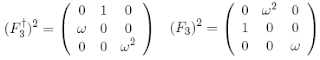occasional meanderings in physics' brave new world

## Friday, October 24, 2008

### CKM Rules IV

Let's consider how the double Fourier transform acts on CKM type operators. Since the three dimensional operator $F_{3}$ cycles the 3d MUBs, and these operators (along with their 2-circulant duals) may be used to form a basis for a $3 \times 3$ matrix $A$, then it follows that $F^{4}$ (the fourth iteration of the transform) is the identity. This is made explicit by the following.

Observe thatThis 2-circulant transformation acts on a general $3 \times 3$ matrix $A$ to giveand we see that the quadruple transform returns the matrix $A$. For the measured CKM values, the triple transform must therefore return the inverse of the $SU(2) \times U(1)$ form of the matrix.

Now the two dimensional operator $F_{2}$, which forms the other component of the neutrino mixing matrix, acts on the Pauli basis for $SU(2)$ to simply cycle the three basis operators. Therefore, the triple application of the MNS transform $(F_{3}F_{2})$ to the CKM matrix yields an inverse also. It follows that

$U T^{3} = T^{3} C$

where $U$ is the CKM group element, $C$ the CKM matrix, and $T$ is the tribimaximal mixing matrix $T = F_{3}F_{2}$. Thus $T$ is a very special operator that acts this way for any (magic) unitary matrix.Kea said...

P.S. Carl, I still haven't found the fairy fields lying under any bushes.

October 24, 2008 3:56 PMMatti Pitkänen said...

A report about this morning's little step of progress is out of topic but in spirit with general category fanatism;-).

I proved that fusion algebras indeed form an operad.

*The local improvement of measurement resolution means a replacement of point to which algebra element is assigned with a set of points and replacement of algebra element with a collection of new algebra elements.

*The notion of measurement resolution allows to guess what are the new structure constants.

*There exists a natural projection homomorphism to the original algebra fixed by the notion of finite measurement resolution.

*What results is a fractal operad. From any elementary building block algebra for N point one can construct new ones by improving measurement resolution at various points by adding any number of points inside little disk around it and thus replacing the element with building block algebra corresponding to some N.

*A collection of trees describes what I interpret as disk operad: at any node of tree arbitrary number of branches can appear. Fractal structure means that any element of any fusion algebra can be replaced by any fusion algebra.

Incredible as it sounds, it seems that I have solved symplectic QFT exactly! Not a trivial achievement since symplectic QFT resolves the UV divergence problem of QFTs by coding the absence of local divergences to the nilpotency condition phi^2=0.

See my blog.

October 24, 2008 5:58 PMKea said...

Thanks, Matti. I am sure this is relevant to the much more simpler exercise that we are engaged in. Much to do.

October 24, 2008 6:01 PM